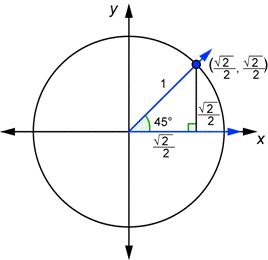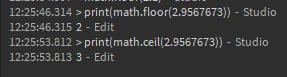# How to understand math.cos,sin,tan,acos,asin,atan

I know there’s a topic similar, What can I do with these asin, acos, atan, sin, cos, tan but I don’t understand it pretty much, I already know this are trigonomic (or something like that) functions, but, for example

The answers people give, sometimes are really complicated, or I don’t get it pretty much, I speak spanish too. so it’s difficult, I want if possible, short answers like for example:

math.abs() – returns all numbers in positive, absolute
math.clamp(x, min, max) returns min if x is < min and max if x is > max
math.floor() rounds down to whole number
math.ceil() rounds up to whole number
math.sin() – ?
math.cos() –
math.tan() – ?

This one I don’t think it’s really needed but well, I mostly want to learn about sin,cos,tan.

math.acos() ?
math.asin() ?
math.atan() ?

## Note, I might be AFK a while, I will mark solution later19 Likes

I think math.floor rounds down and math.ceil rounds up instead of it being the other way around

8 Likes

Well, the truth is that you need to take geometry and linear algebra/pre-calc to truly understand the strength of these built-ins not only programmatically, but also in the real world. A one-sentence explanation for `math.sin(x)` would be like this:

“returns the sine of the x parameter where x is assumed to be a number in radians”
BUT
This doesn’t really mean anything to anyone under the age of 13-15 unless they’ve had early exposure to geometry/linear algebra/pre-calc. What is a radian? What’s the relationship between radians and degrees? What is pi? How does trigonometry work in planar math? What is slope?

I’m not going to explain in depth these oscillating wave functions or trigonometric principles, but I wish you luck!

For the most part, if I am trying to do 3D animations with CFrames I use these math built-ins, but I don’t use them for anything else on the Roblox platform at the moment.

11 Likes

I was also having the same issues with math-related scripts but yeah my friend told me I’ll learn about everything shortly.

1 Like

IIRC all the sine, cosine and tangent functions are running on radians and if not, degrees. Requires some thinking about the unit circle. Referenced by this image.

7 Likes

Sine (`math.sin`) is the ratio of the opposite and hypotenuse sides of a triangle based off of an angle (θ1) in standard position.
Cosine and tangent are other ratios, and the other ones (`math.a[xxx]()`) are just their inverse.For example, the sine of 45° is sqrt(2)/2.
It may sound overwhelming, but you’ll learn about trigonometric functions eventually - I just learned the math recently.

5 Likes

Sine, cosine, tangent, arcosine, arcsine, and arctangent are all trigonemtric functions. In short, they are mainly used to describe the relation between the length of the sides of a right triangle and the angle between the hypotenuse (the longest side of the right triangle) and it’s adjacent side (the other side of the triangle next to the angle). Trigonometry itself centers around the use of them, and they are very important functions in math.
As for practical uses for the functions themselves, it helps to look visually at graphs of them. If you dont know how to interpret these graphs, you can image the x-axis as the value you put into the function and the y-value as what you would get back, so for example, the sine graph visualizes the possible results of:

``````local y = math.sin(x);
``````

Some of my favorite practical uses of these functions are to model oscillations, or a number going back and forth between two different values. Let’s say we want to move a part up and down, back and forth smoothly. We can do that easily using sine:

``````local part = script.Parent;
local position = part.Position;
game:GetService("RunService").Stepped:Connect(function(currentTime)
end)
``````

The result:

Here’s another interesting oscillation, this time using tangent. I imagine this might look nice for a UI element.

``````local part = script.Parent;
local position = part.Position;
game:GetService("RunService").Stepped:Connect(function(currentTime)
end)
``````

They’re fun to use to make interesting movement.

21 Likes

This is not true, floor rounds up and ceil round down.

math.floor(x) removes any decimals the number has, so the integer is left.
math.ceil(x) removes any decimals too but adds 1 to the integer.
For example calling math.floor(2.845) will result in 2, which is rounding down.
But calling math.ceil(2.155) will result in 3, which is rounding up.
And for rounding to integers, you can use math.floor(x + 0.5) or math.ceil(x - 0.5)
Or for rounding to any decimal use this:

``````--[[
x: number (Number to round)
d: number (Amount of decimals to round to, use negative if you want to round to tens, hundreds, etc)
]]--
function round(x, d)
d = d or 0
local f = math.pow(10, d)
return math.floor(x * f + 0.5) / f
end
``````

Hi, @varjoy, glad that you want to learn these functions! And yes, they not are easy at the start but with a bit time you can learn from these. Sinus, Cosinus and Tangent are trigonometric functions, and as @mobiusInfinity say, they are used in right triangles to get the length of a side. And as the most (i think) functions they also have they inverses: Arc-Sinus, Arc-Cosinus and Arc-Tangent. With these you can hold a side as parameter and get with it a angle. So, i know that speaking most not help, so i will try to show you a example:

I tried here to get the angle between the player‘s head LookVector and the LookVector of the camera for rotating the head to the camera, to do this i needed the InverseTrigonometric functions. Here you can see that i have now 2 Vectors.

So, Theta is the angle i want get, so i can rotate the head. If i remember what @mobiusInfinity say:

There are 3 Main words, that you need to know: Hypotenuse (the longest side), Opposite (the opposite lies opposite a given angle) and the Adjacent (The adjacent is the side that lies at the given angle but is not the hypotenuse). The Khan Academy can help you with this if you want to go more in deep. Now that you know this, we can use SOH (Sinus = Opposite/Hypotenuse), CAH (Cosinus = Adjacent/Hypotenuse) and TOA (Tangent = Opposite/Adjacent).

I have my triangle (you can immagine you that we closes the two vectors to create a triangle) and know my lenght, but still need to know my angle: What now? Now its time for the Inverses (Arc Sinus-Cosinus-Tangent)! I know the Hypotenuse and the Adjacent, so the function we need is the Cosinus (CAH, Adjacent and Hypotenuse), however we dont need the normal Cosinus function, rather the Arc-cosinus (acos = cos^-1), as we are searching a angle. With this we know that:

And if we try to write this in Lua:

``````local Adjacent = game.Players.LocalPlayer.Head.CFrame
local Hypotenuse = workspace.CurrentCamera.CFrame
--The both are CFrames, not Vectors, but it should help you to understand

local FinalVector = Adjacent:ToObjectSpace(Hypotenuse).LookVector --We need to make the 2 vectors relative, ldk as without this it dont work. Still Remember we are using 2 CFrames and the function only takes 1 Parameter

local Theta = math.acos(FinalVector)
``````
32 Likes

No actually math.floor does round down and math.ceil rounds up.

Example:

``````print(math.floor(23.13))
``````

It would print 23 and:

``````print(math.ceil(23.13))
``````

would print 24.

Also it is in the name. Floor is at the bottom and ceiling is at the top.
If you don’t believe me, try it yourself instead of spreading misinformation.

1 Like

Hey! All of you helped me SOOO much, I don’t know who deserves the solution… this is complicated.

I guess I will focus on trigonometry now!

1 Like

Here’s a great tutorial by Brackeys that explains it pretty well:

11 Likes

It’s in the name of their functions. Imagine yourself at home, where is the floor?
Exactly, you’re standing on it. Meaning, the floor is below you (down) and the floor function rounds down. Now where is the ceiling (ceil)? If you look up, you notice it’s on top of you. Meaning, it’s above you and the ceil function rounds up.

I also confused those 2 functions before but after taking a closer look at their names, it already becomes obvious that the floor function represents the floor and the ceil function represents the ceiling.

What?
Lmao no, absolubtly wrong.

How am I wrong? If you have a number which is “2.9175392” for example, using the math.floor function will make it 2. And then using the math.ceil function, it will make it 3. You can try that using the Command bar. Open the Command bar and the Output window, type `print(math.floor(2.9175392))` and the output will print 2. Doing the same thing but using the ceil function and it will print 3. Meaning the analogy I brought up earlier is correct.2 Likes

It’s ok if you can’t script, but make sure to do some research before replying such nonsense. You are supposed to give your point of view in that way.

1 Like

Ypou can’t compare math.floor with looking at your house floor… how does that makes even sense?
The “analogy” is not even a proper example, you’re talking about a house floor. Both are well explained on devhub, check it out.

How doesn’t it make any sense? If a house floor is on the bottom, that means the math.floor function goes down. And indeed, like the devhub says, it rounds a number down. And a house ceiling is on top, which means the math.ceil function goes up. And again, like the devhub says, it rounds a number up. Your point?

3 Likes